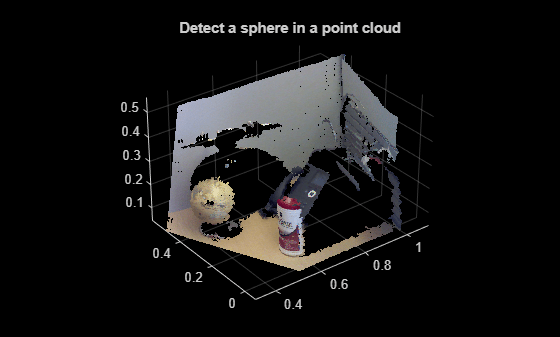Documentation

# sphereModel class

Object for storing a parametric sphere model

## Syntax

```model = sphereModel(params) ```

## Description

Object for storing a parametric sphere model

## Construction

`model = sphereModel(params)` constructs a parametric sphere model from the 1-by-4 `params` input vector that describes a sphere.

### Input Arguments

expand all

Sphere parameters, specified as a 1-by-4 scalar vector. This input specifies the `Parameters` property. The four parameters [a,b,c,d] satisfy the equation for a sphere:

`${\left(x-a\right)}^{2}+{\left(y-b\right)}^{2}+{\left(z-c\right)}^{2}={d}^{2}$`

## Properties

expand all

Sphere model parameters, stored as a 1-by-4 vector. These parameters are specified by the `params` input argument. The four parameters [a,b,c,d] satisfy the equation for a sphere:

`${\left(x-a\right)}^{2}+{\left(y-b\right)}^{2}+{\left(z-c\right)}^{2}={d}^{2}$`

Center of the sphere, stored as a 1-by-3 vector [xc,yc,zc] that specifies the center coordinates of the sphere.

Radius of sphere, stored as a scalar value.

## Methods

 plot Plot sphere in a figure window

## Examples

collapse all

`load('object3d.mat');`

Display point cloud.

```figure pcshow(ptCloud) xlabel('X(m)') ylabel('Y(m)') zlabel('Z(m)') title('Detect a sphere in a point cloud')```Set the maximum point-to-sphere distance (1cm), for sphere fitting.

`maxDistance = 0.01;`

Set the region of interest to constrain the search.

```roi = [-inf, 0.5; 0.2, 0.4; 0.1, inf]; sampleIndices = findPointsInROI(ptCloud, roi);```

Detect the globe in the point cloud and extract it.

`model = pcfitsphere(ptCloud, maxDistance, 'SampleIndices', sampleIndices);`

Plot the sphere.

```hold on plot(model)```